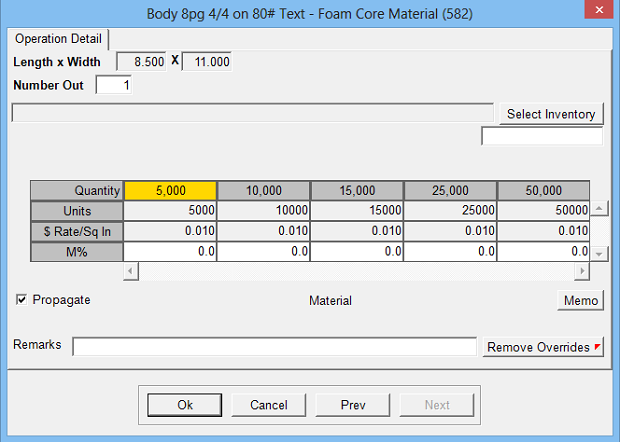Estimates the cost of material by the square inch.  Commonly used for Large Format estimates.

Operation Setup Requirements:
1. Description
2. Rate: Material Cost per square inch
3. Sell \$ use: Markup % (recommended) or alternate sell rate
4. Calc: 3
5. Sub Key: Material
6. Rate Per M: Makes \$ Rate per thousand square inches
8. Set Units to Quantity option
9. Round up Square Inch Calculation Option
10. Set size to: Zero (editable), Form Run Size or Form Finish SizeEstimate Specification Input Prompts:

1. Length x Width (inches)
2. Units (not editable if set to Quantity)
3. Markup %
4. Remarks
5. MemoCalculation:

Length x Width = Square inches per Unit

Square Inches per Unit x Units = Total Square Inches

Total Square Inches x Rate = Cost \$

Cost \$ x Markup % = Markup \$

Cost \$ + Markup \$ = Sell \$